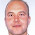# Learning Clojure

## Wednesday, September 25, 2013

### Efficiency and Progress II : Behold, C

```/* A guest language on this blog. A welcome, please, for C */

/* The reason that I am off on this particular one at the moment is
because I recently waited 3 hours for a clojure program to
terminate, after about a day trying to get it to run at all. */

/* When it became apparent that it was not going to finish any time
soon, I hacked together an answer in C, using what should have been
a less efficient algorithm that I had come up with while
waiting. */

/* That program took about 15 minutes to write and 65 seconds to run,
and got the correct answer. */

/* That comparison is entirely unfair to both clojure and C in all
sorts of ways, but if I am going to spend time getting clojure to
run at C-ish speeds, I need to know what I should be aiming for. */

/* This program is what I am using as a comparison for (reduce + (map + _ _ )) */

/* To make sure that clever compilers and runtimes aren't doing any
sneaky dead-code elimination, it is actually doing some sort of
computation. But it is mainly mapping and reducing. Lots.*/

#include<stdio.h>

#define N 1000000

int a[N];
int b[N];

int main(void)
{
int i, count;
long long sum=0;

for (i=0; i< N; i++) {
a[i]=i;
}

for(count=0; count<1000; count++){
for (i=0; i< N; i++) {
b[i]+=a[i];
}

for (i=0; i< N; i++) {
sum+=b[i];
}
}

printf("sum=%lli\n", sum);
}

/* gcc -std=gnu99 -Ofast efficiencyandprogress.c -o efficiencyandprogress && time ./efficiencyandprogress */
/* sum=250249749750000000 */

/* real 0m16.053s */
/* user 0m15.992s */
/* sys  0m0.032s */

/* So it looks as though adding one array to another and then adding up all the values in an array takes about 16ms in total.*/
/* That's 16ns per array entry which looks sort of OK on my little netbook, which boast an Intel Atom CPU N455 running at 1.66GHz with a 512kb*/

/* I'm hoping there's enough complexity here that the compiler actually has to run the program rather than taking short cuts*/

/* But just as a check, here's the code running with gcc in 'do exactly what I say so I can debug it' mode.*/

/* gcc -std=gnu99 -O0 efficiencyandprogress.c -o efficiencyandprogress && time ./efficiencyandprogress */
/* sum=250249749750000000 */

/* real 0m27.850s */
/* user 0m27.692s */
/* sys  0m0.060s */

/* This produces a small constant factor speedup, as expected if the two versions are doing the same work. */
```

1.Thx again for your most interesting blog posts !

You might find it interesting to compile your C code with «gcc -S speed.c -O4 -o speed.S -march=native -ftree-vectorize -ftree-vectorizer-verbose=2 --verbose-asm» and look at the .S file.
I'd be quite surprised if the JIT could leverage the vector instructions like gcc does, unfortunately.

Cheers,

Bernard

1.Cool! Thanks Bernand, I didn't know you could do that. That doubles the speed again.
My gcc doesn't have an O4, but does seem to have an Ofast. At any rate, the march=native is the trick.

gcc -std=gnu99 efficiencyandprogress.c -Ofast -march=native -o efficiencyandprogress && time ./efficiencyandprogress
sum=250249749750000000

real 0m8.606s
user 0m8.572s
sys 0m0.024s

2.Even faster to recognize that this is an arithmetic series.
The first term is: 999999*500000 (the sum of the numbers from 0 to 1000000 - it is just another arithmetic series)
The last term is: 1000 * 999999*500000
The number of terms: 1000
So the sum is: 1000 * 1001 * 999999*500000 / 2 = 250249749750000000

http://en.wikipedia.org/wiki/Arithmetic_progression#Sum

3."the sum of the numbers from 0 to 1000000" -> it is from 0 to 999999 of course

4.And if it is just about compiler microbenchmarks, this small haskell program runs in 0.4s in my machine:
main = print \$ sum \$ take 1001 [0,sum [0..999999]..]

I made a version more similar to the C one, and it runs the same speed (0.3-0.4s):
main = print \$ sum \$ take 1000 \$ zipWith (*) [1..] (repeat (sum [0..999999]))

With -O0 it is 1.3s

5.I've managed to reach 140s running time with this code, this one finally forces the program to really make the 1000000 long iteration O(1000) times:
main = print \$ sum \$ take 1000 \$ map sum \$ zipWith (\x y -> map (*x) y) [1..] (repeat [0..999999])

6.This runs in 0.3 sec on my pc:
#include

#define N 1000000

int b[N];

int main(void)
{
unsigned int i, count;
long long sum=0;

for(count=0; count<1000; count++){
for (i=0; i< N; i++) {
b[i]+=i;
sum+=b[i];
}

}

printf("sum=%lli\n", sum);
}

time ./fast
sum=250249749750000000

real 0m0.360s
user 0m0.360s
sys 0m0.000s

the version with multiple loops is slower, i think because of cache effects